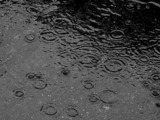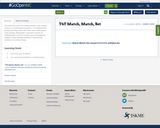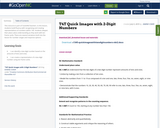Updating search results...

# 5 Results

View
Selected filters:
• quantityConditional Remix & Share Permitted
CC BY-NC
Rating
0.0 stars
Rating
0.0 stars
Subject:
Mathematics
Material Type:
Full Course
Provider:
Pearson
11/02/2020Conditional Remix & Share Permitted
CC BY-NC
Rating
0.0 stars

Ratios

Type of Unit: Concept

Prior Knowledge

Students should be able to:

Calculate with whole numbers up to 100 using all four operations.
Understand fraction notation and percents and translate among fractions, decimal numbers, and percents.
Interpret and use a number line.
Use tables to solve problems.
Use tape diagrams to solve problems.
Sketch and interpret graphs.
Write and interpret equations.

Lesson Flow

The first part of the unit begins with an exploration activity that focuses on a ratio as a way to compare the amount of egg and the amount of flour in a mixture. The context motivates a specific understanding of the use of, and need for, ratios as a way of making comparisons between quantities. Following this lesson, the usefulness of ratios in comparing quantities is developed in more detail, including a contrast to using subtraction to find differences. Students learn to interpret and express ratios as fractions, as decimal numbers, in a:b form, in words, and as data; they also learn to identify equivalent ratios.

The focus of the middle part of the unit is on the tools used to represent ratio relationships and on simplifying and comparing ratios. Students learn to use tape diagrams first, then double number lines, and finally ratio tables and graphs. As these tools are introduced, students use them in problem-solving contexts to solve ratio problems, including an investigation of glide ratios. Students are asked to make connections and distinctions among these forms of representation throughout these lessons. Students also choose a ratio project in this part of the unit (Lesson 8).

The third and last part of the unit covers understanding percents, including those greater than 100%.

Students have ample opportunities to check, deepen, and apply their understanding of ratios, including percents, with the selection of problems in the Gallery.

Subject:
Mathematics
Statistics and Probability
Provider:
PearsonConditional Remix & Share Permitted
CC BY-NC
Rating
0.0 stars

Students use percents greater than 100% to solve problems about rainfall, revenue, snowfall, and school attendance.Key ConceptsPercents greater than 100% are useful in making comparisons between the values of a single quantity at two points in time. When a later value is more than 100% of an earlier value, it means the quantity has increased over time. This percent comparison can be used to find unknown values, whether the earlier or later value is unknown.Goals and Learning ObjectivesUnderstand the meaning of a percent greater than 100% in real-world situations.Use percents greater than 100% to interpret situations and solve problems.

Material Type:
Lesson Plan
Author:
Pearson
11/02/2020Conditional Remix & Share Permitted
CC BY-NC-SA
Rating
0.0 stars

This resource is part of Tools4NCTeachers.

Each student receives a card denoting a quantity 1-10. Students form groups by finding others who have cards containing the same quantity.

Being able to represent numbers in multiple ways is a part of number sense. Kindergarten students should develop flexibility in representing numbers in a variety of ways.

Subject:
Mathematics
Material Type:
Lesson
Lesson Plan
11/06/2019Conditional Remix & Share Permitted
CC BY-NC-SA
Rating
0.0 stars

This resource is part of Tools4NCTeachers.

In this lesson, students build fluency with recognizing and creating representations of numbers within 100. Students deepen their place value understanding as they work with ten frame cards. There are several variations built into the lesson for number ranges and response options.

Subject:
Mathematics
Material Type:
Activity/Lab
Lesson
Lesson Plan